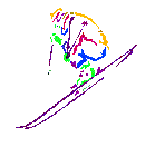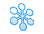Search:

# Snow in New Zealand

## Other## Related

(:template first:)

 {=\$:Name}? {=\$:chair6x} {=\$:chair4x} {=\$:chair4} {=\$:chair3} {=\$:chair2} {=\$:liftTbar} {=\$:liftplatter} {=\$:liftropehand} {=\$:liftcarpet} {=\$:area} {=\$:base} {=\$:top} {=\$:drop} {=\$:terrain} {=\$:aspect} {=\$:opens} {=\$:closes} {=\$:url}(approve sites)